## Fit Data with a Shallow Neural Network

Neural networks are good at fitting functions. In fact, there is proof that a fairly simple neural network can fit any practical function.

Suppose, for instance, that you have data from a health clinic. You want to design a network that can predict the percentage of body fat of a person, given 13 anatomical measurements. You have a total of 252 example people for which you have those 13 items of data and their associated percentages of body fat.

You can solve this problem in two ways:

It is generally best to start with the app, and then use the app to automatically generate command-line scripts. Before using either method, first define the problem by selecting a data set. Each of the neural network apps has access to many sample data sets that you can use to experiment with the toolbox (see Sample Data Sets for Shallow Neural Networks). If you have a specific problem that you want to solve, you can load your own data into the workspace. The next section describes the data format.

Note

To interactively build, visualize, and train deep learning neural networks, use the Deep Network Designer app. For more information, see Get Started with Deep Network Designer.

### Defining a Problem

To define a fitting (regression) problem for the toolbox, arrange a set of input vectors (predictors) as columns in a matrix. Then, arrange a set of responses (the correct output vectors for each of the input vectors) into a second matrix. For example, you can define a regression problem with four observations, each with two input features and a single response, as follows:

```predictors = [0 1 0 1; 0 0 1 1]; responses = [0 0 0 1]; ```

The next section shows how to train a network to fit a data set, using the Neural Net Fitting app. This example uses an example data set provided with the toolbox.

### Fit Data Using the Neural Net Fitting App

This example shows how to train a shallow neural network to fit data using the Neural Net Fitting app.

Open the Neural Net Fitting app using `nftool`.

`nftool`Select Data

The Neural Net Fitting app has example data to help you get started training a neural network.

To import example body fat data, select Import > Import Body Fat Data Set. You can use this data set to train a neural network to estimate the body fat of someone from various measurements. If you import your own data from file or the workspace, you must specify the predictors and responses, and whether the observations are in rows or columns.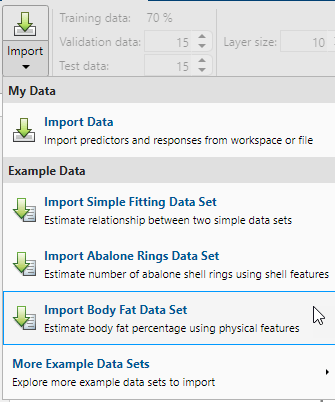Information about the imported data appears in the Model Summary. This data set contains 252 observations, each with 13 features. The responses contain the body fat percentage for each observation.Split the data into training, validation, and test sets. Keep the default settings. The data is split into:

• 70% for training.

• 15% to validate that the network is generalizing and to stop training before overfitting.

• 15% to independently test network generalization.

For more information on data division, see Divide Data for Optimal Neural Network Training.

Create Network

The network is a two-layer feedforward network with a sigmoid transfer function in the hidden layer and a linear transfer function in the output layer. The Layer size value defines the number of hidden neurons. Keep the default layer size, 10. You can see the network architecture in the Network pane. The network plot updates to reflect the input data. In this example, the data has 13 inputs (features) and one output.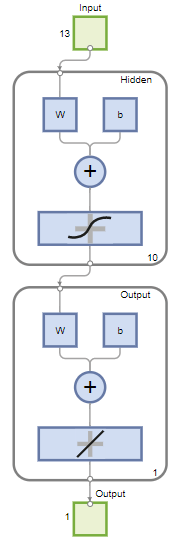Train Network

To train the network, select Train > Train with Levenberg-Marquardt. This is the default training algorithm and the same as clicking Train.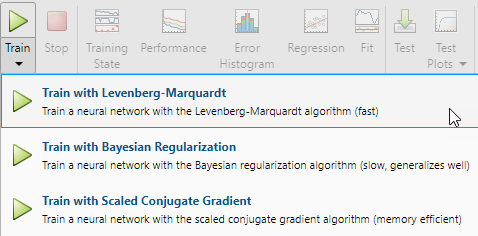Training with Levenberg-Marquardt (`trainlm`) is recommended for most problems. For noisy or small problems, Bayesian Regularization (`trainbr`) can obtain a better solution, at the cost of taking longer. For large problems, Scaled Conjugate Gradient (`trainscg`) is recommended as it uses gradient calculations which are more memory efficient than the Jacobian calculations the other two algorithms use.

In the Training pane, you can see the training progress. Training continues until one of the stopping criteria is met. In this example, training continues until the validation error increases consecutively for six iterations ("Met validation criterion").Analyze Results

The Model Summary contains information about the training algorithm and the training results for each data set.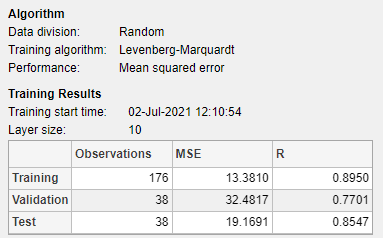You can further analyze the results by generating plots. To plot the linear regression, in the Plots section, click Regression. The regression plot displays the network predictions (output) with respect to responses (target) for the training, validation, and test sets.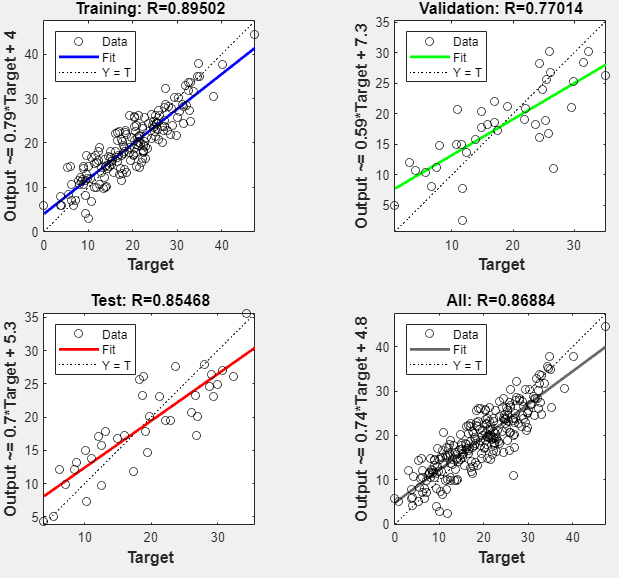For a perfect fit, the data should fall along a 45 degree line, where the network outputs are equal to the responses. For this problem, the fit is reasonably good for all of the data sets. If you require more accurate results, you can retrain the network by clicking Train again. Each training will have different initial weights and biases of the network, and can produce an improved network after retraining.

View the error histogram to obtain additional verification of network performance. In the Plots section, click Error Histogram.The blue bars represent training data, the green bars represent validation data, and the red bars represent testing data. The histogram provides an indication of outliers, which are data points where the fit is significantly worse than most of the data. It is a good idea to check the outliers to determine if the data is poor, or if those data points are different than the rest of the data set. If the outliers are valid data points, but are unlike the rest of the data, then the network is extrapolating for these points. You should collect more data that looks like the outlier points and retrain the network.

If you are unhappy with the network performance, you can do one of the following:

• Train the network again.

• Increase the number of hidden neurons.

• Use a larger training data set.

If performance on the training set is good but the test set performance is poor, this could indicate the model is overfitting. Reducing the number of neurons can reduce the overfitting.

You can also evaluate the network performance on an additional test set. To load additional test data to evaluate the network with, in the Test section, click Test. The Model Summary displays the additional test results. You can also generate plots to analyze the additional test data results.

Generate Code

Select Generate Code > Generate Simple Training Script to create MATLAB code to reproduce the previous steps from the command line. Creating MATLAB code can be helpful if you want to learn how to use the command line functionality of the toolbox to customize the training process. In Fit Data Using Command-Line Functions, you will investigate the generated scripts in more detail.Export Network

You can export your trained network to the workspace or Simulink®. You can also deploy the network with MATLAB Compiler™ tools and other MATLAB code generation tools. To export your trained network and results, select Export Model > Export to Workspace.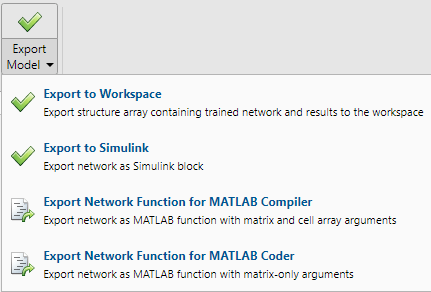### Fit Data Using Command-Line Functions

The easiest way to learn how to use the command-line functionality of the toolbox is to generate scripts from the apps, and then modify them to customize the network training. As an example, look at the simple script that was created in the previous section using the Neural Net Fitting app.

```% Solve an Input-Output Fitting problem with a Neural Network % Script generated by Neural Fitting app % Created 15-Mar-2021 10:48:13 % % This script assumes these variables are defined: % % bodyfatInputs - input data. % bodyfatTargets - target data. x = bodyfatInputs; t = bodyfatTargets; % Choose a Training Function % For a list of all training functions type: help nntrain % 'trainlm' is usually fastest. % 'trainbr' takes longer but may be better for challenging problems. % 'trainscg' uses less memory. Suitable in low memory situations. trainFcn = 'trainlm'; % Levenberg-Marquardt backpropagation. % Create a Fitting Network hiddenLayerSize = 10; net = fitnet(hiddenLayerSize,trainFcn); % Setup Division of Data for Training, Validation, Testing net.divideParam.trainRatio = 70/100; net.divideParam.valRatio = 15/100; net.divideParam.testRatio = 15/100; % Train the Network [net,tr] = train(net,x,t); % Test the Network y = net(x); e = gsubtract(t,y); performance = perform(net,t,y) % View the Network view(net) % Plots % Uncomment these lines to enable various plots. %figure, plotperform(tr) %figure, plottrainstate(tr) %figure, ploterrhist(e) %figure, plotregression(t,y) %figure, plotfit(net,x,t)```

You can save the script and then run it from the command line to reproduce the results of the previous training session. You can also edit the script to customize the training process. In this case, follow each step in the script.

#### Select Data

The script assumes that the predictor and response vectors are already loaded into the workspace. If the data is not loaded, you can load it as follows:

`load bodyfat_dataset`

This command loads the predictors `bodyfatInputs` and the responses `bodyfatTargets` into the workspace.

This data set is one of the sample data sets that is part of the toolbox. For information about the data sets available, see Sample Data Sets for Shallow Neural Networks. You can also see a list of all available data sets by entering the command `help nndatasets`. You can load the variables from any of these data sets using your own variable names. For example, the command

```[x,t] = bodyfat_dataset; ```

will load the body fat predictors into the array `x` and the body fat responses into the array `t`.

#### Choose Training Algorithm

Choose training algorithm. The network uses the default Levenberg-Marquardt algorithm (`trainlm`) for training.

`trainFcn = 'trainlm'; % Levenberg-Marquardt backpropagation. `

For problems in which Levenberg-Marquardt does not produce as accurate results as desired, or for large data problems, consider setting the network training function to Bayesian Regularization (`trainbr`) or Scaled Conjugate Gradient (`trainscg`), respectively, with either

```net.trainFcn = 'trainbr'; net.trainFcn = 'trainscg';```

#### Create Network

Create a network. The default network for function fitting (or regression) problems, `fitnet`, is a feedforward network with the default tan-sigmoid transfer function in the hidden layer and linear transfer function in the output layer. The network has a single hidden layer with ten neurons (default). The network has one output neuron because there is only one response value associated with each input vector.

```hiddenLayerSize = 10; net = fitnet(hiddenLayerSize,trainFcn);```

Note

More neurons require more computation, and they have a tendency to overfit the data when the number is set too high, but they allow the network to solve more complicated problems. More layers require more computation, but their use might result in the network solving complex problems more efficiently. To use more than one hidden layer, enter the hidden layer sizes as elements of an array in the `fitnet` command.

#### Divide Data

Set up the division of data.

```net.divideParam.trainRatio = 70/100; net.divideParam.valRatio = 15/100; net.divideParam.testRatio = 15/100;```

With these settings, the predictor vectors and response vectors are randomly divided, with 70% for training, 15% for validation, and 15% for testing. For more information about the data division process, see Divide Data for Optimal Neural Network Training.

#### Train Network

Train the network.

```[net,tr] = train(net,x,t); ```

During training, the training progress window opens. You can interrupt training at any point by clicking the stop button.Training finished when the validation error increased consecutively for six iterations. If you click in the training window, a plot of the training errors, validation errors, and test errors appears, as shown in the following figure. In this example, the result is reasonable because of the following considerations:

• The final mean-square error is small.

• The test set error and the validation set error have similar characteristics.

• No significant overfitting has occurred by epoch 13 (where the best validation performance occurs).#### Test Network

Test the network. After the network has trained, you can use it to compute the network outputs. The following code calculates the network outputs, errors, and overall performance.

```y = net(x); e = gsubtract(t,y); performance = perform(net,t,y)```
```performance = 16.2815```

It is also possible to calculate the network performance only on the test set by using the testing indices, which are located in the training record. For more information, see Analyze Shallow Neural Network Performance After Training.

```tInd = tr.testInd; tstOutputs = net(x(:,tInd)); tstPerform = perform(net,t(tInd),tstOutputs)```
```tstPerform = 20.1698```

#### View Network

View the network diagram.

```view(net) ```#### Analyze Results

Analyze the results. To perform a linear regression between the network predictions (outputs) and the corresponding responses (targets), click in the training window.The output tracks the responses well for training, testing, and validation sets, and the R-value is over 0.87 for the total data set. If even more accurate results were required, you could try any of these approaches:

• Reset the initial network weights and biases to new values with `init` and train again.

• Increase the number of hidden neurons.

• Use a larger training data set.

• Increase the number of input values, if more relevant information is available.

• Try a different training algorithm (see Training Algorithms).

In this case, the network response is satisfactory, and you can now put the network to use on new data.

#### Next Steps

To get more experience in command-line operations, try some of these tasks:

Also, see the advanced script for more options, when training from the command line.

Each time a neural network is trained can result in a different solution due to random initial weight and bias values and different divisions of data into training, validation, and test sets. As a result, different neural networks trained on the same problem can give different outputs for the same input. To ensure that a neural network of good accuracy has been found, retrain several times.

There are several other techniques for improving upon initial solutions if higher accuracy is desired. For more information, see Improve Shallow Neural Network Generalization and Avoid Overfitting.